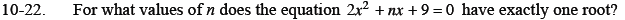Home > CCA2 > Chapter Ch10 > Lesson 10.1.1 > Problem10-22

10-22.

For what values of n does the equation 2x2 + nx + 9 = 0 have exactly one root? Homework Help ✎This equation can be solved using the Quadratic Formula.
When does the Quadratic Formula yield exactly one solution/root?

The Quadratic Formula yields exactly one solution when the value inside the square root is zero.

b2 − 4ac = 0 or in this case, n2 − 4(2)(9) = 0.
Solve for n.